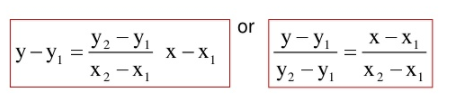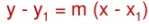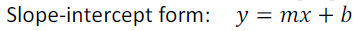# Two Point Form, Point Slope Form, Slope Intercept Form, Standard Form , Equation of Line Formulas & Calculator# Three Forms Of Equation Of A Straight Line Calculator

An online slope equations of a st.line calculation# Formula : Equations of A Straight Line

## Two-Point Form

••
• ### Point-Slope Form

••
• ## Point Intercept Form

••
• ## Lines Parallel to Axes

• Equation of straight line parallel to x-axis at a distance 'a' is y = a and equation of straight line parallel to y-axis at distance 'b' is x = b.
•# Solved Examples

Example: Solve the equation of a line with a slope of 5 and a y-intercept of (0, -7).

Since m = 5 and (0, -7) is the y-intercept, b = -7, then substituting into the form y = mx + b

Equation of a Line is 5x -y -7 , can write as y = 5x - 7